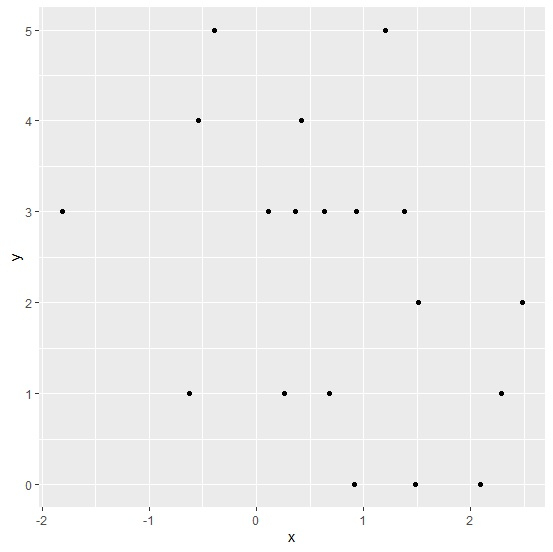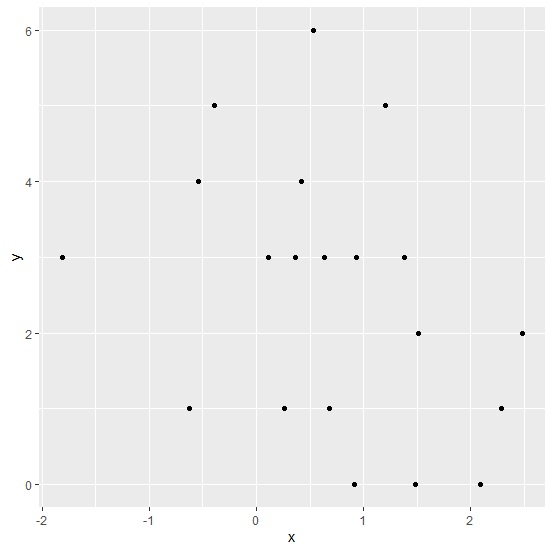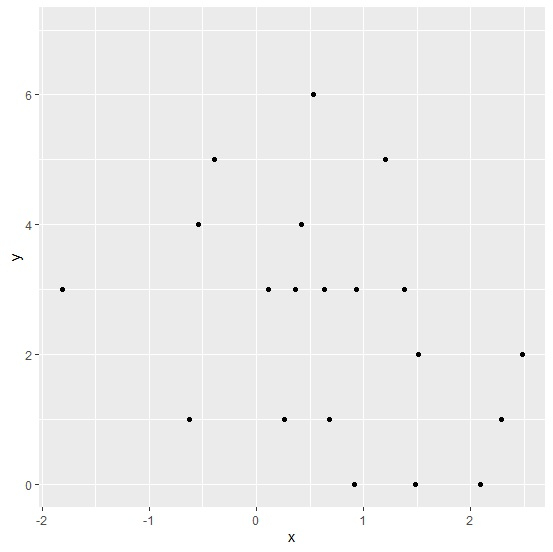# How to deal with warning “removed n rows containing missing values” while using ggplot2 in R?

The warning “removed n rows containing missing values” occurs when we incorrectly specify the range of the values for X-axis or Y-axis. We can this range in ggplot function using scale_x_continuous(limits=c(?,?)) for x axis and scale_y_continuous(limits=c(?,?)) for y axis. If the range will be larger than the actual data range then there will be no warning otherwise, we will get the warning for the number of missing values.

## Example

Consider the below data frame −

> set.seed(2)
> x<-rnorm(20,0.5)
> y<-rpois(20,2)
> df<-data.frame(x,y)

> library(ggplot2)

Creating the plot with Y-axis limits from 0 to 5−

> ggplot(df,aes(x,y))+
+ geom_point()+
+ scale_y_continuous(limits=c(0,5))
Warning message:
Removed 1 rows containing missing values (geom_point).

## OutputHere, we got a warning for 1 row with missing value. Now let’s create a plot with increasing one value for Y-axis −

> ggplot(df,aes(x,y))+
+ geom_point()+
+ scale_y_continuous(limits=c(0,6))

## OutputHere, we can see that 6 is added on the Y-axis and there are no warnings. We can also increase the limit depending on our need as shown below −

> ggplot(df,aes(x,y))+
+ geom_point()+
+ scale_y_continuous(limits=c(0,7))

## Output